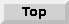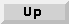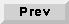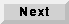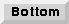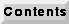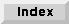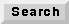## 9.4 An overview of PN stars

The "open-palette" command in pigi ("O") will open a checkbox window that you can use to open the standard palettes in all the installed domains. For the PN domain, the star library is small enough that it is easily contained entirely in one palette, shown in figure 9-1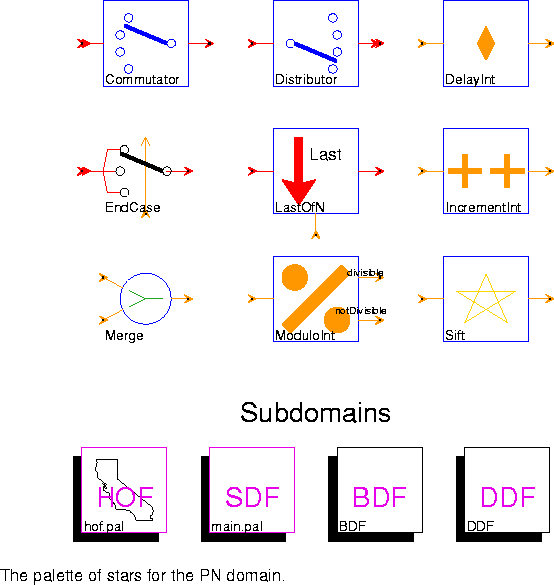Many of these stars are re-implementations of similarly named stars in the SDF and DDF domains. These implementations take advantage of the multi-threaded nature of execution in the PN domain.

`Commutator` Takes N input streams (where N is the number of inputs) and synchronously combines them into one output stream. It consumes B particles from an input (where B is the blockSize), and produces B particles on the output, then it continues by reading from the next input. The first B particles on the output come from the first input, the next B particles from the next input, etc.
`Distributor` Takes one input stream and splits it into N output streams, where N is the number of outputs. It consumes B input particles, where B = blockSize, and sends them to the first output. It consumes another B input particles and sends them to the next output, etc.
`DelayInt` An initializable delay line.
`EndCase` Depending on the "control" particle, consume a particle from one of the data inputs and send it to the output. The value of the control particle should be between zero and N-1, where N is the number of data inputs.
`LastOfN` Given a control input with integer value N, consume N particles from the data input and produce only the last of these at the output.
`Merge` Merge two increasing sequences, eliminating duplicates.
`IncrementInt` Increment the input by a constant.
`ModuloInt` Divides the input stream into a stream of numbers divisible by N and another stream of numbers that are not divisible by N.